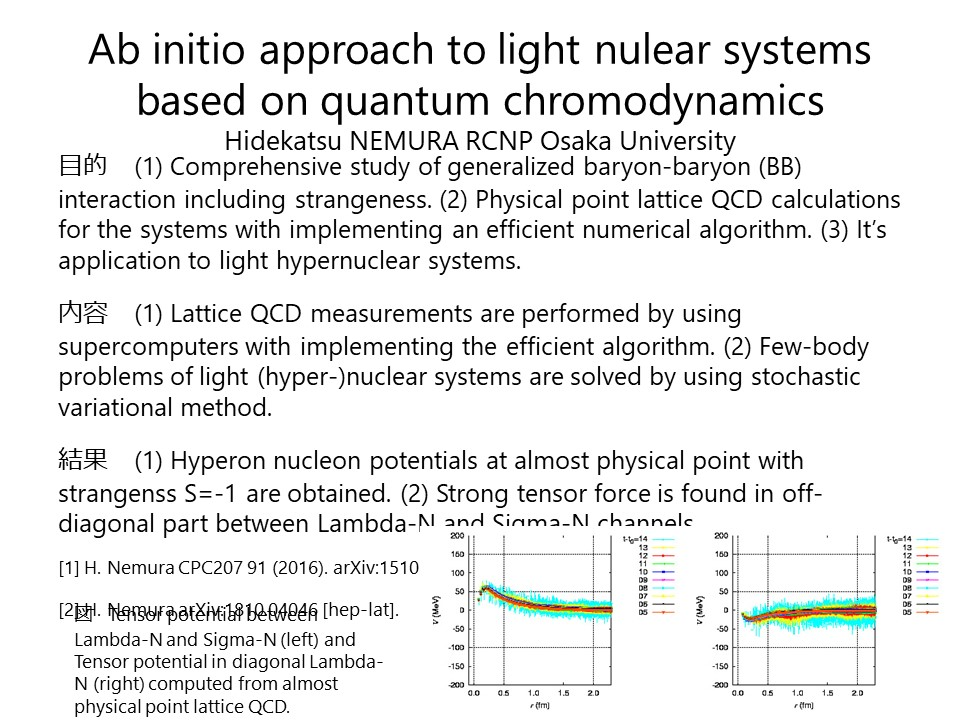Ab initio approach to light nuclear systems based on quantum chromodynamicsAuthors：Hidekatsu NEMURA

Affiliation： RCNP Osaka University

Abstract：Comprehensive study of generalized baryon-baryon interaction including strangeness is one of the important subject of nuclear physics. In order to obtain a complete set of isospin-base baryon interactions, we perform a large scale lattice QCD calculation with almost physical quark masses corresponding to $(m_{\pi}, m_{K})\approx(146,525)$~MeV and large volume $(La)^4=(96a)^4\approx(8.1~{\rm fm})^4$. A large number of Nambu-Bethe-Salpeter correlation functions from nucleon-nucleon to $\Xi\Xi$ are calculated simultaneously. For the moment, we focus on the strangeness S=-1 channels of the hyperon interactions by means of HAL QCD method. We find that the tensor force between Lambda-Nucleon and Sigma-Nucleon is stronger than the tensor force in the Lambda-Nucleon diagonal part.

• 根村英克, "格子QCDによる一般化核力研究と$\Lambda$N、$\Sigma$N ポテンシャル", 日本物理学会第76回年次大会, 2021年3月12-15日, オンライン開催.